# Ex 13.6 NCERT Solutions- Surface Areas and Volumes Class 9 Notes | EduRev

## Class 9 : Ex 13.6 NCERT Solutions- Surface Areas and Volumes Class 9 Notes | EduRev

The document Ex 13.6 NCERT Solutions- Surface Areas and Volumes Class 9 Notes | EduRev is a part of the Class 9 Course Class 9 Mathematics by VP Classes.
All you need of Class 9 at this link: Class 9

Note: For the following questions, assume π = (22/7), unless stated otherwise

Question 1. The circumference of the base of a cylindrical vessel is 132 cm and its height is 25 cm.
How many litres of water can it hold? (1000 cm3 = 1 l)
Solution:
Let the base radius of the cylindrical vessel be ‘r’ cm.
∴ Circumference = 2πr
⇒ 2πr = 132                             [∵ Circumference = 132 cm]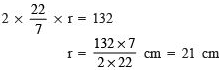∵ Height of the vessel = 25 cm
∴ Volume = πr2 x h                               [∵ Volume of a cylinder = πr2h]
= (22/7) (21)2 x 25 cm3

= (22/7) x 21 x 21 x 25 cm3
= 22 x 3 x 21 x 25 cm3
= 34650 cm
∵ Capacity of the vessel = Volume of the vessel
∴ Capacity of cylindrical vessel = 34650 cm
Since 1000 cm= 1 litre
⇒ 34650 cm3 = (34650/1000) litres = 34.65 l

Question 2. The inner diameter of a cylindrical wooden pipe is 24 cm and its outer diameter is 28 cm. The length of the pipe is 35 cm. Find the mass of the pipe, if 1 cm3 of wood has a mass of 0.6 g.
Solution:
Here, Inner diameter of the cylindrical pipe = 24 cm
⇒ Inner radius of the pipe (r) = (24/2) cm = 12 cm
Outer diameter of the pipe = 28 cm
⇒ Outer radius of the pipe (R) = (28/2)cm = 14 cm
Length of the pipe (h) = 35 cm
∵ Inner volume of the pipe = πr2h
Outer volume of the pipe = πr2h
∴ Amount of wood (volume) in the pipe = Outer volume – Inner volume

= πR2h - πr2h

= πh(R2-r2)

= πh(R+r)(R-r) [ ∵ a2 - b2 = (a+b)(a-b)]

= 22/7 x 35 x (14+12) x (14-12) cm3

= 22 x 5 x 26 x 2 cm3

Mass of the wood in the pipe = [Mass of wood in 1 m3 of wood] x [Volume of wood in the pipe]
= [0.6g] x [22 x 5 x 26 x 2] cm3
= (6/10)x 22 x 10 x 26 g = 6 x 22 x 26 g
= 3432 g = (3432/1000)= 3.432 kg                        [∵ 1000 g = 1 kg]
Thus, the required mass of the pipe is 3.432 kg.

Question 3. A soft drink is available in two packs (i) a tin can with a rectangular base of length 5 cm and width 4 cm, having a height of 15 cm and (ii) a plastic cylinder with circular base of diameter 7 cm and height 10 cm. Which container has greater capacity and by how much?
Solution:
For rectangular pack: Length (l) = 5 cm
Breadth (b) = 4 cm Height (h) = 15 cm
∴ Volume = l x b x h = 5 x 4 x 15 cm3
= 300 cm3
⇒ Capacity of the rectangular pack = 300 cm3                     ...(1)
For cylindrical pack: Base diameter = 7 cm
⇒ Radius of the base (r) = (7/2)cm
Height (h) = 10 cm

∴ Volume = πr2h = (22/7) x (7/2)2 x 10 cm3
= (22/7) x (7/2) x (7/2) x 10 cm3
= 11 x 7 x 5 cm3 = 385 cm3
⇒ Volume of the cylindrical pack = 385 cm3                     ...(2)
From (1) and (2),
we have 385 cm3 – 300 cm3 = 85 cm3
⇒ The cylindrical pack has the greater capacity by 85 cm3.

Question 4. If the lateral surface of a cylinder is 94.2 cmand its height is 5 cm, then find: (i) radius of its base (ii) its volume. (Use π = 3.14)
Solution:
Height of the cylinder (h) = 5 cm Let the base radius of the cylinder be ‘r’.
(i) Since lateral surface of the cylinder = 2 πrh
But lateral surface of the cylinder = 94.2 cm2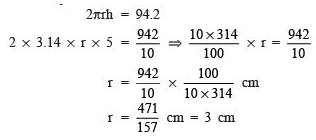Thus, the radius of the cylinder = 3 cm

(ii) Volume of a cylinder = πr2h
⇒ Volume of the given cylinder = 3.14 x (3)2 x 5 cm3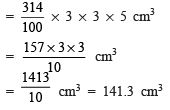Thus, the required volume = 141.3 cm3

Question 5. It costs  2200 to paint the inner curved surface of cylindrical vessel 10 m deep. If the cost of painting is at the rate of  20 per m2; find: (i) inner curved surface of the vessel (ii) radius of the base (iii) capacity of the vessel.
Solution:
(i) To find inner curved surface

Total cost of painting = ₹ 2200

Rate of painting = ₹ 20 per m2

∴     Area =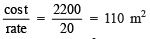⇒ Inner curved surface of the vessel = 110 m2

(ii) To find radius of the base Let the base radius of the cylindrical vessel.
∵ Curved surface of a cylinder = 2 πrh
∴                  2πrh = 110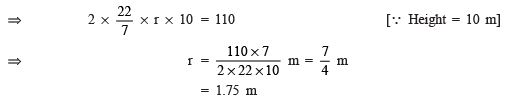⇒ The required radius of the base = 1.75 m

(iii) To find the capacity of the vessel

Since, volume of a cylinder = πr2h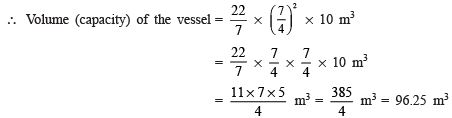Since, 1 m3 = 1000000 cm3 = 1000 l = 1 kl
∴ 96.5 m3 = 96.5 kl
Thus, the required volume = 96.25 kl

Question 6. The capacity of closed cylindrical vessel of height 1 m is 15.4 litres. How many square metres of metal sheet would be needed to make it?
Solution:
Capacity of the cylindrical vessel = 15.4 l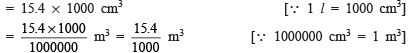⇒ Volume of the vessel = (15.4/1000)m3
Height of the vessel = 1m Let ‘r’ metres be the radius of the base of the vessel
∴ Volume = πr2h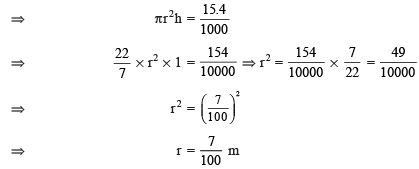Now, total surface area of the cylindrical vessel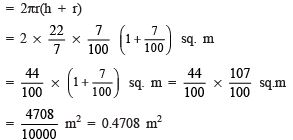Thus, the required sheet = 0.4708 m2

Question 7. A lead pencil consists of a cylinder of wood with a solid cylinder of graphite filled in the interior. The diameter of the pencil is 7 mm and the diameter of graphite is 1 mm. If the length of the pencil is 14 cm, find the volume of the wood and that of the graphite.
Solution:
Since, 10 mm = 1cm
∴ 1 mm = (1/10) cm
For graphite cylinder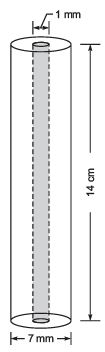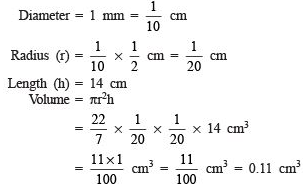Thus, the required volume of the graphite = 0.11 cm
For the pencil Diameter of the pencil = 7 mm = (7/10)cm
∴ Radius of the pencil (R) = (7/20) cm

Height of the pencil (h) = 14 cm

Volume of the pencil =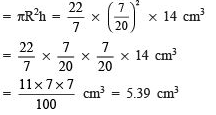Volume of the wood Volume of the wood = [Volume of the pencil] – [Volume of the graphite]
= 5.39 cm3 – 0.11 cm3 = 5.28 cm
Thus, the required volume of the wood is 5.28 cm3.

Question 8. A patient in a hospital is given soup daily in a cylindrical bowl of diameter 7 cm. If the bowl is filled with soup to a height of 4 cm, how much soup the hospital has to prepare daily to serve 250 patients?
Solution:
The bowl is cylindrical.
Diameter of the base = 7 cm
⇒ Radius of the base (r) = (7/3) cm
Height (h) = 4 cm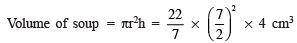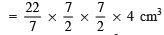= 11 x 7 x 2 cm3 = 154 cm3
i.e. Volume of soup in a bowl = 154 cm3
⇒ Volume of soup in 250 bowls = 250 x 154 cm3
= 38500 cm3

= 38500 / 100 liters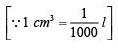Thus, the hospital needs to prepare 38.5 litres of soup daily for 250 patients.

VOLUME OF A RIGHT CIRCULAR CONE

REMEMBER
Volume of a cone = (1/3) πr2h, where ‘r’ is its base radius and ‘h’ is its height.

Offer running on EduRev: Apply code STAYHOME200 to get INR 200 off on our premium plan EduRev Infinity!

132 docs

,

,

,

,

,

,

,

,

,

,

,

,

,

,

,

,

,

,

,

,

,

;